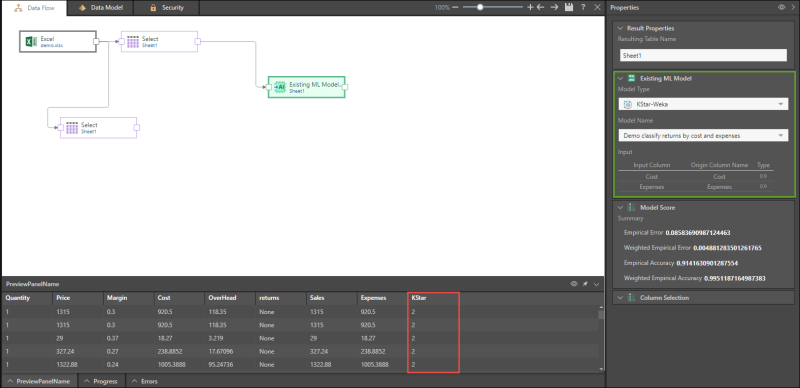# Machine Learning Examples

## Bins

Use this node to group a specified numeric column into value ranges. Select a numeric column as the input column, then choose the method of binning (Standard Deviation or Percentile), the total number of bins, and name the resulting column.

In the example below, the given input column is Price, and the chosen method of binning is Standard Deviation, with 4 bins. In the column Binning of Price Range (designated in red), each row is sorted into one of the four bins, based on the Price column.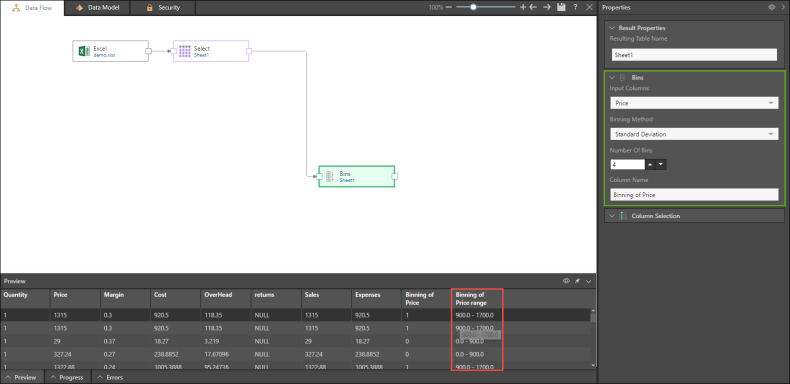## Outlier

Use this node to determine whether or not an item is an outlier. Under Input Columns, select a numeric columns. Next select the standard deviation, choose whether to add a new outlier column, or replace outliers in the input column with the mean, extrapolation, or median.

In the following example, the input given is the Price column, and a standard deviation of 2 is selected. The results are displayed in a new column called Outlier Column (in red).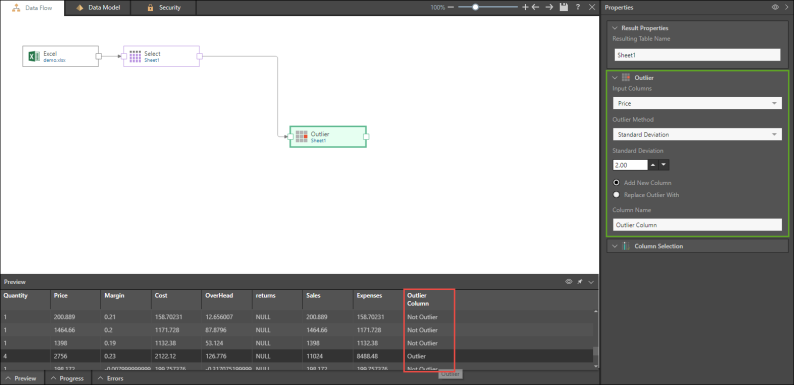## Clustering (Canopy)

Use the Canopy Weka node to sort large datasets into clusters. The input columns must be numeric. Once you've selected the input columns, select the Running Process Type, and name the new column. The Running Process Type determines the number of rows in the preview.

In the example below, four input columns were selected and were sorted into two clusters (0 and 1) in the new Canopy column that was produced.## Classification (KNN)

Based on the given inputs and outputs, KNN Weka can be used to make predictions such as the likelihood of somebody making a purchase based on their income, age, and previous purchases. This node requires a numeric input columns and a nominal target column. Select the required input columns and then the required target column (under Output Classifier), then select the minimum number of nearest neighbors, the running process type, and name the new column.

In the below example, the given inputs are cost, expenses, and margin, and the output classifier is returns. The column produced will indicate the predicted number of returns based on the input columns.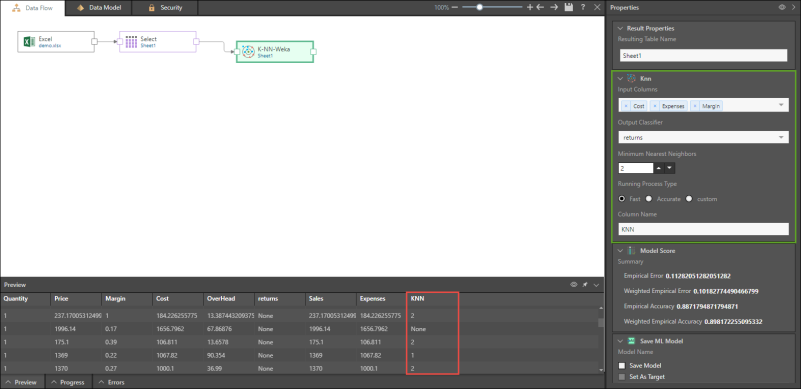## Save Model and Set as Target

A number of machine learning algorithms may be saved as a machine learning model. Select Save Model to choose this option.

You will also have the option to set the model as the target. This enables you to use a given machine learning algorithm to compare datasets.

In the example below, the KStar Weka algorithm is used to predict returns by cost and expenses, and the result is saved as a model and set as the target (in red).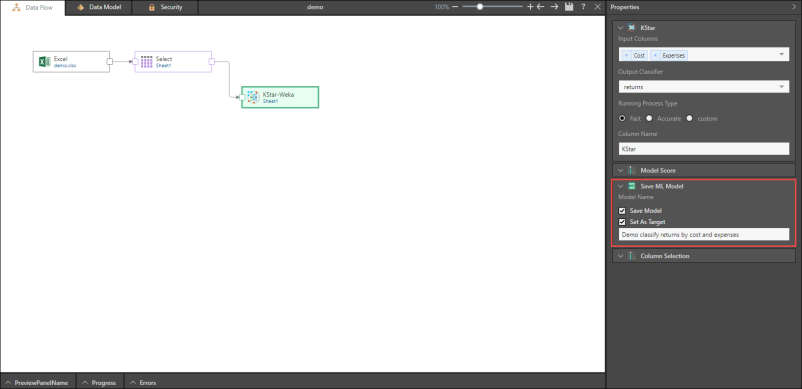Here, the saved Machine Learning model is added to the ETL. Note that the data columns in the machine learning model must be in the same format as the columns in the table to which it is connected.

This is useful for building a model over existing data that is well known and understood, and later using the model on new data. For example, it is possible to build machine learning models that predict customers’ purchasing behaviors according to customers’ inquiring behaviors in the last 2 years, and then use this data on new customers, predicting how much, when, and what they will purchase according to their inquiries.